# MP Board Class 7th Maths Solutions Chapter 5 Lines and Angles Ex 5.2

## MP Board Class 7th Maths Solutions Chapter 5 Lines and Angles Ex 5.2

Question 1.
State the property that is used in each of the following statements?
(i) If a||b, then ∠1 = ∠5.
(ii) If ∠4 = ∠6, then a||b.
(iii) If ∠4 + ∠5 = 180°,then a||b.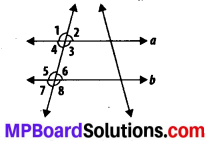Solution:
(i) Corresponding angles property
(ii) Alternate interior angles property
(iii) Interior angles on the same side of the transversal are supplementary.Question 2.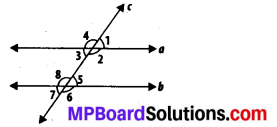(i) the pairs of corresponding angles.
(ii) the pairs of alternate interior angles.
(iii) the pairs of interior angles on the same side of the transversal.
(iv) the vertically opposite angles.
Solution:
(i) ∠1 and ∠5; ∠2 and ∠6;
∠3 and ∠7; ∠4 and ∠8
(ii) ∠2 and ∠8; ∠3 and ∠5
(iii) ∠2 and ∠5; ∠3 and ∠8
(iv) ∠1 and ∠3; ∠2 and ∠4;
∠5 and ∠7; ∠6 and ∠8Question 3.
In the adjoining figure, p||q. Find the unknown angles.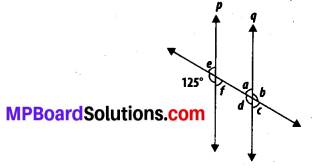Solution:
∠d = 125° (Corresponding angles)
∠e = 180° – 125° = 55° (Linear pair)
∠f = ∠e = 55° (Vertically opposite angles)
∠c = ∠f = 55° (Corresponding angles)
∠a = ∠e – 55° (Corresponding angles)
∠b = ∠d = 125° (Vertically opposite angles)Question 4.
Find the value of x in each of the following figures l ||m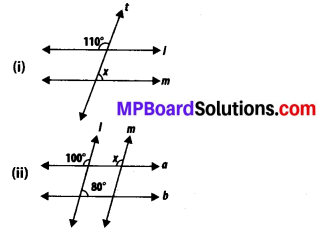Solution:
(i) We have,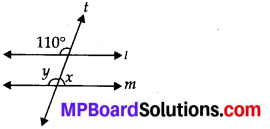y = 110° (Corresponding angles)
x + y = 180° (Linear pair)
⇒ x = 180° -110° = 70°

(ii) x = 100° (Corresponding angles)

Question 5.
In the given figure, the arms of two angles are parallel. If ∠ABC = 70°, then find
(i) ∠DGC
(ii) ∠DEF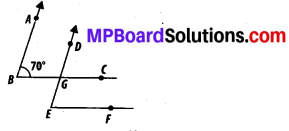Solution:
(i) Consider that AB||DG and a transversal line BC is intersecting them.
∴ ∠DGC = ∠ABC (Corresponding angles)
⇒ ∠DGC = 70°

(ii) Consider that BC 11 EF and a transversal line DE is intersecting them.
∴ ∠DEF = ∠DGC (Corresponding angles)
⇒ ∠DEF = 70°Question 6.
In the given figures below, decide whether l is parallel to m.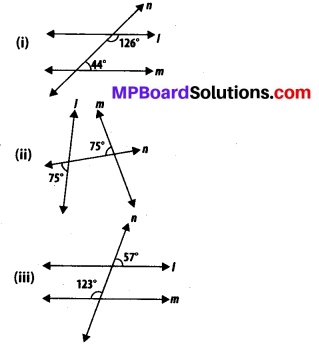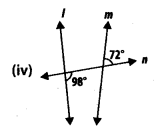Solution:
(i) Consider two lines l and m and a transversal line n which is intersecting them. Sum of the interior angles on the same side of transversal = 126° + 44° = 170°.
As the sum of interior angles on the same side of the transversal is not 180°, therefore, 1 is not parallel to m.

(ii) We have,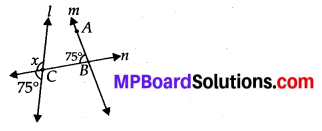x + 75° = 180°(Linear pair on line l)
⇒ x = 180° – 75° = 105°
For l and m to be parallel to each other, corresponding angles (∠ABC and x) should be equal. However, here their measures are 75° and 105°. Hence, the lines l and m are not parallel to each other.

(iii) We have,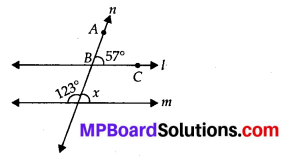x + 123° = 180° (Linear pair on line m)
⇒ x = 180° – 123° = 57°
For l and m to be parallel to each other, corresponding angles (∠ABC and x) should be equal. However their measures are equal to 57°. Hence, l||m.

(iv) We have,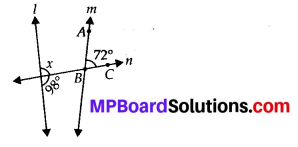98° + x = 180°(Linear pair on line l)
⇒ x = 180° – 98° = 82°
For l and m to be parallel to each other, corresponding angles (∠ABC and x) should be equal. However, their measures are 72° and 82°. Hence, the lines l and m are not parallel to each other.